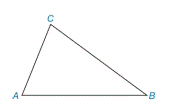Chapter 2.4, Problem 2E### Elementary Geometry for College St...

6th Edition
Daniel C. Alexander + 1 other
ISBN: 9781285195698

#### Solutions

Chapter
Section### Elementary Geometry for College St...

6th Edition
Daniel C. Alexander + 1 other
ISBN: 9781285195698
Textbook Problem
31 views

# In Exercise 1 to 4, refer to Δ A B C . On the basis of the information given, determine the measure of the remaining angle(s) of the triangle. m ∠ B = 39 ° and m ∠ C = 82 °To determine

To find:

The measure of A.

Explanation

Given:

The given statement is,

mB=39° and mC=82°

Figure (1)

Calculation:

In a triangle, the sum of the measures of the interior angles is 180°.

The given statement is,

mB=39° and mC=82°.

The sum of the measures of the interior angles is 180°

### Still sussing out bartleby?

Check out a sample textbook solution.

See a sample solution

#### The Solution to Your Study Problems

Bartleby provides explanations to thousands of textbook problems written by our experts, many with advanced degrees!

Get Started

#### Solve the equations in Exercises 126. x+4x+1+x+43x=0

Finite Mathematics and Applied Calculus (MindTap Course List)

#### x3a+b3

Applied Calculus for the Managerial, Life, and Social Sciences: A Brief Approach

#### True or False: converges.

Study Guide for Stewart's Multivariable Calculus, 8th